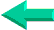### This is an excerpt from Pierre Leclerc (www.excel-vba.com)

The 2 Most Important

Excel Functions AND
Formulas

SUMPRODUCT

AND

INDEX/MATCH

## Lesson 5: Excel Spreadsheets  Information Functions (17)

In annex 5 you have found a description of all 17 Excel functions in the "Information" category. Below is the list of the 2 most useful ones. See more on the very powerful ISERROR fonction.

 Functions What it Does ISERROR Returns TRUE if the value is any error value CELL Returns information about the formatting, location, or contents of a cell

Examples of Basic Information Formulas

CELL, MID, FIND

If you want the name and path of the active spreadsheet to be entered automatically in a cell, use the formula: =CELL("filename" )
if you want only the filename use :
=MID(CELL("filename" ,A1),FIND("[" ,CELL("filename" ,A1))+1,FIND("]" ,CELL("filename" ,A1))-FIND("[" ,CELL("filename",A1))-1)

ISERROR/ISNA

When a formula refers to a cell in which you have another formula, always use the ISERROR function to avoid trashing the last formula with a " #DIV/0" or a " #VALUE" or a " #N/A" .
=IF(ISERROR(B1/A1),"",(B1/A1))
if the value of cell A1 is 0, the cell in which you have put the above formula will be empty and not carry a value of #DIV/0.
=IF(ISERROR(B1/A1),0,(B1/A1))
if the value of cell A1 is 0, the value of the cell in which you have put the above formula will be 0 and not #DIV/0.
I also use the ISERROR rather then the ISNA function when I work with
INDEX/MATCH.

IF, ISNUM, LEFT  and MID

In UK all postal codes start by a prefix of one or two letters. My correspondent wanted a formula to extract the prefixes so he could make a list of them. With the postal codes in column one the following formula in column 2 would do the job.
=IF(ISNUMBER(MID(A1,2,1)*1),LEFT(A1,1),LEFT(A1,2))
Depending on the number of characters in the prefix the formula should return the first  character from the left or the first 2  characters from the left: LEFT(A1,1) or LEFT(A1,2)
Before any of these solutions is applied we must check if there are one or two letters at the beginning of the postal code. To do so we will check if the second character
MID(A1,2,1)  is a number. The problem here is that any character from a text string is consider as a letter by Excel. Postal codes, serial numbers and others that include a letter or are formatted as text  are text by nature . So we multiply the second character by 1. If the character is a digit  to begin with it becomes a number but if it is a letter it doesn't: ISNUMBER(MID(A1,2,1)*1).

SUMPRODUCT  ¦  INDEX/MATCH  ¦  SUBTOTAL  ¦  ISERROR  ¦  HLOOKUP  ¦  LOOKUP  ¦  VLOOKUP
AVERAGEIF  ¦  AVERAGEIFS ¦  COUNTIF  ¦  COUNTIFS  ¦  SUMIF  ¦  SUMIFS  ¦  IFERRORBack home